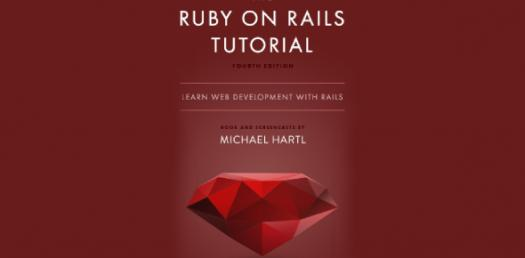# Book M Ch 4 Test

24 Questions | Total Attempts: 157Settings.

• 1.
Place a checkmark beside all of the things that would be considered work.
• A.

Typing

• B.

Holding a pencil

• C.

Studying

• 2.
The unit of measure for power is
• A.

Newton

• B.

Joule

• C.

Watt

• 3.
The unit of measure for work is
• A.

Newton

• B.

Joule

• C.

Watt

• 4.
The unit of measure for force is
• A.

Newton

• B.

Joule

• C.

Watt

• 5.
The unit of measure for distance is
• A.

Pascal

• B.

Meter

• C.

Second

• 6.
The unit of measure for time is
• A.

Pascal

• B.

Meter

• C.

Second

• 7.
The unit of measure for pressure is
• A.

Pascal

• B.

Meter

• C.

Second

• 8.
A device that makes work easier by changing the size or direction of the applied force.
• A.

Work

• B.

Machine

• C.

Efficiency

• 9.
This causes the output work of a machine to be less than the input force
• A.

• B.

Work

• C.

Friction

• 10.
The rate at which work is being done.
• A.

Power

• B.

Wheel an Axles

• C.

Efficiency

• 11.
A moving inclined plane
• A.

Wedge

• B.

Wheel and axle

• C.

Screw

• 12.
A simple machine has _____ movement (s).
• A.

One

• B.

Two

• C.

Three

• 13.
Two rigidly attached wheels that rotate together
• A.

Inclined plane

• B.

Wheel and axle

• C.

Pulley

• 14.
A sloped surface
• A.

Wedge

• B.

Inclined plane

• C.

Screw

• 15.
Exertion of a force on an object that produces motion in the direction of the force
• A.

Power

• B.

• C.

Work

• 16.
The force a machine puts out
• A.

Input force

• B.

Output force

• 17.
The force you exert on a machine
• A.

Input force

• B.

Output force

• 18.
The pivot point of a lever.
• A.

Machine

• B.

Pulley

• C.

Fulcrum

• 19.
An inclined plane wrapped around a shaft.
• A.

Wedge

• B.

Screw

• C.

Wheel and axle

• 20.
A grooved wheel that redirects force (changes the direction)using a rope.
• A.

Pulley

• B.

Wheel and axle

• C.

Wedge

• 21.
To lift a crate, a pulley system exerts a force of 3,500 N. Find the mechanical advantage of the pulley system if the input force is 70 N.  Put ONLY the numeric value.
• 22.
You do 100 J of work in pulling out a nail with a claw hammer. If the hammer does 50 J of work, what is the hammer's efficiency? ___% (numeric value only)
• 23.
A teacher does 1450 J of work in 6 s. How much power did he use? ____W (numeric value only).
• 24.
As you push a lawn mover, the horizontal force is 700 N. If you push the mower a distance of 900 M, how much work do you do? ____J (numeric value only, make sure you use a comma).
Related TopicsBack to top